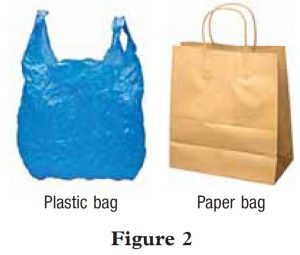# Summative Practice 3 (Question 1 & 2) – Form 5 Science (KSSM) Chapter 3

Question 1:
Figure 1 shows an experiment to study the level of water pollution in different water samples.Table 1 shows the time taken for the methylene blue solution to decolourise in the different water samples in Figure 1.(a) State one hypothesis for this experiment.

(b) State the variables in this experiment.
(i) Constant variable
(ii) Manipulated variable

(c) Based on Table 1, which water sample is the most polluted?

(d) Based on this experiment, state the relationship between the water pollution level and the time taken for methylene blue solution to decolourise.

(a) River water is the most polluted water sample compared to tap water, distilled water and pond water.

(b)(i) Volume of water
(b)(ii) Type of water sample

(c) River water

(d) The higher the level of water pollution, the shorter the time taken for the methylene blue solution to decolourise.

Question 2:
Figure 2 shows two types of bags, which are, plastic bag and paper bag.(a) Which of the bags shown in Figure 2 is more environmental-friendly
(b) Give one reason for your answer in question 2(a).
(c) What is microplastic?
(d) Give two examples of plastic products which produce microplastic waste.
(e) State one difference between the carbon footprint and carbon handprint of a product